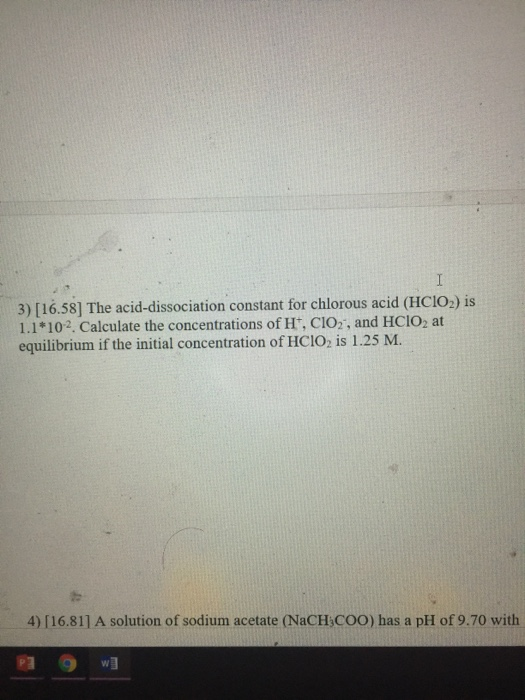# Question & Answer: The acid-dissociation constant for chlorous acid (HClO_2) is 1.1*10^-2. Calculate the…..The acid-dissociation constant for chlorous acid (HClO_2) is 1.1*10^-2. Calculate the concentrations of H^+, ClO_2^-, and HClO_2 at equilibrium if the initial concentration of HClO_2 is 1.25 M. A solution of sodium acetate (NaCH_3COO) has a pH of 9.70 with

3)

HClO2 ——-> H+ + ClO2

Don't use plagiarized sources. Get Your Custom Essay on
Question & Answer: The acid-dissociation constant for chlorous acid (HClO_2) is 1.1*10^-2. Calculate the…..
GET AN ESSAY WRITTEN FOR YOU FROM AS LOW AS \$13/PAGE

1.25 M          0 M     0 M

– x M            + x M   +x M

(1.25 – x) M     x M      x M

Acid dissocaitionc constant = 1.1 * 10-2 = [H+] [ClO2] / [HClO2]

1.1 * 10-2 = [x] [x]/ [1.25 M]

x = 0.117 M

[H+] = 0.117 M

[ClO2] = 0.117 M

[HClO2] = 1.25 M – 0.117 M = 1.133 M